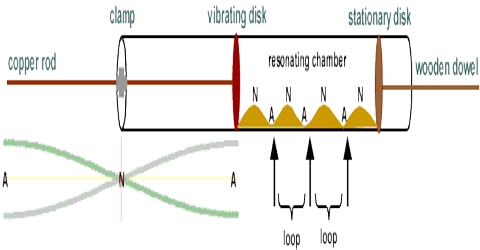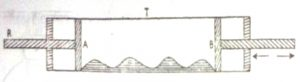Chemistry

# Experimental Determination of γ of Gas in Kundt PrincipleIn the method devised by Kundt it is known as Kundt Principle, the velocity of sound in a gas of pressure, P, and density, ρ, is measured in a simple apparatus. The velocity, v, of the gas is given by the expression

V = √γ.(P/ρ) … … … (1)

The value of γ may be calculated from a knowledge of V.

The apparatus (Figure) consists of a glass tube, T, about a metre long. A thin layer of lycopodium or other fine powder is spread along the tube, which is closed at both ends by corks.Figure: Kundt’s apparatus

The piston, A, is fixed firmly in place and is attached to a glass rod, R. The piston, B, can he moved in and out. The tube is filled with the gas at atmospheric pressure. By gentle strokes on the rod, R. with damp chamois leather the rod is set vibrating. Sound waves are produced which travel in the tube between A and B. The powder forms itself in small heaps at the position of the vibration nodes. The piston, B, is moved in and out to get the best results. The distance between the two heaps is one half the wave length of sound. The tube is now filled with air at the same pressure and the experiment is repeated. We then have;

[Velocity in the gas/Velocity in air] = (γgasair)

γgas and γair are the wavelength of sound in the gas and air respectively.

Thus, knowing the velocity of sound in air the velocity in the gas is calculated.

Again, gasair) = √(γ1 ρ1 / γ2 ρ2)

So, from the ratio of gasair) and knowing γ2 and density ρ2 for air and density ρ1 of the gas, γ for the gas may be determined.